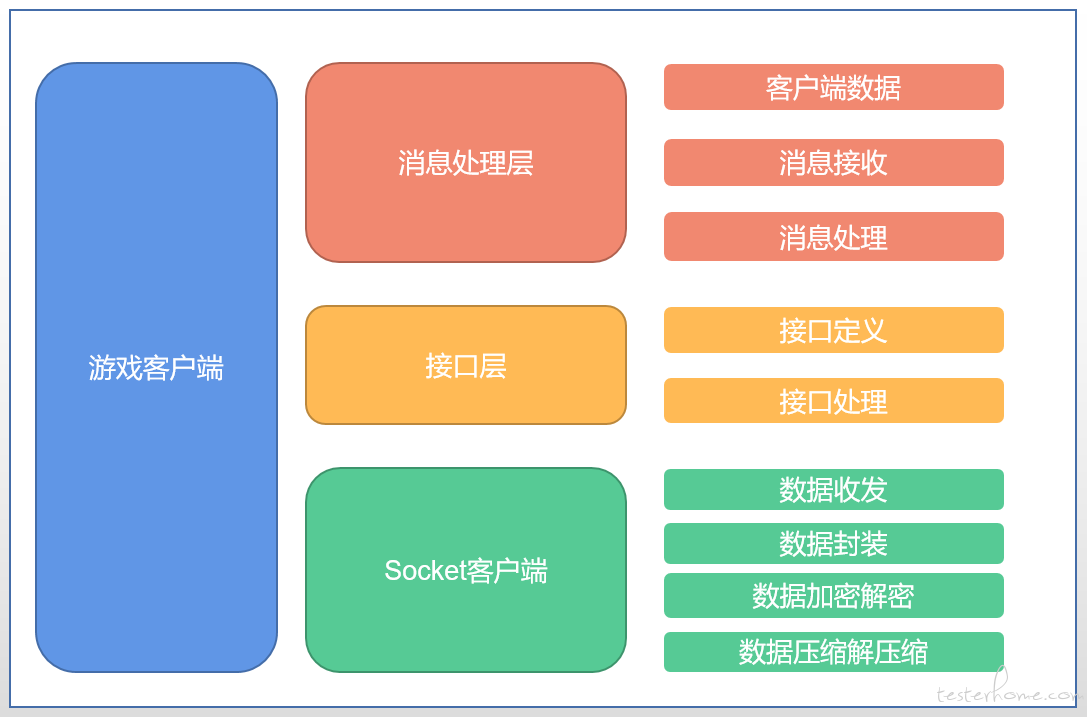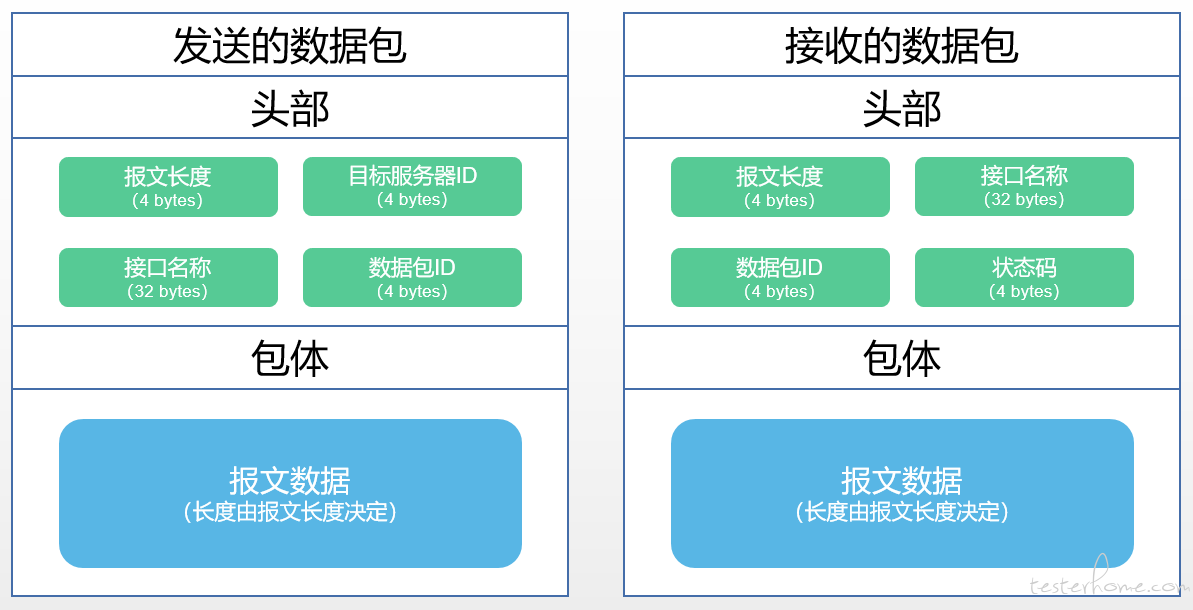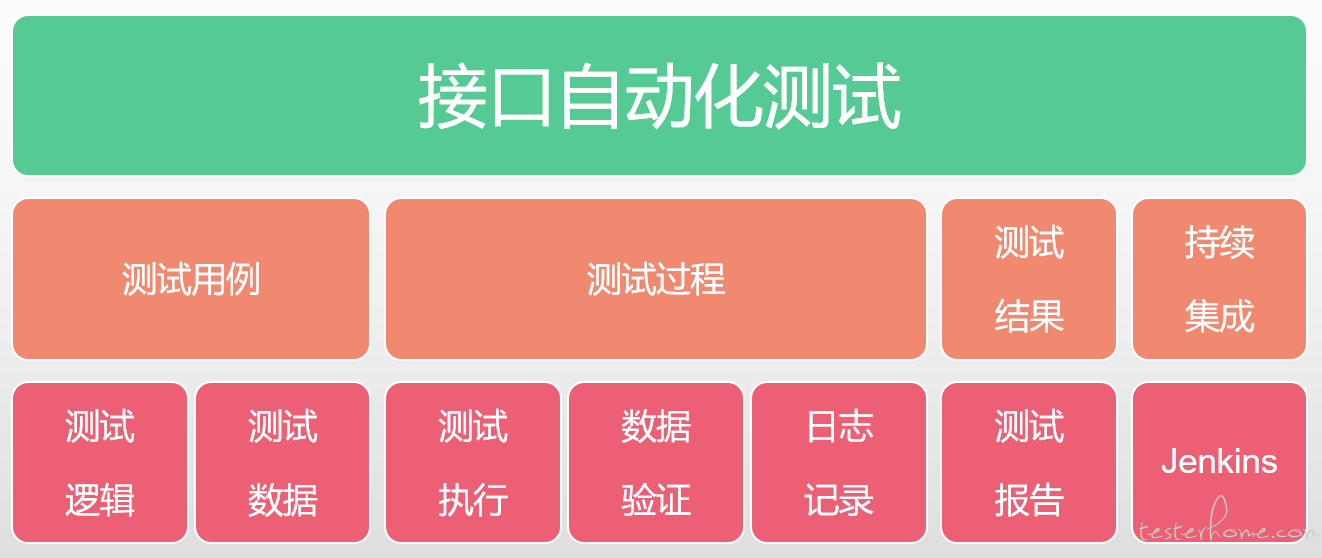# 詹聪聪：游戏接口测试自动化实践

## 2.做一个游戏客户端

### 2.1游戏客户端架构设计### 2.2做一个socket客户端

#### 2.2.1socket客户端

import socket

class Client(object):
def __new__(cls, playerId, game_server, certificate):
cls.playerId = playerId
cls.game_server = game_server
cls.certificate = certificate
cls.cache = b""
return super(Client, cls).__new__(cls)

@classmethod
def server_connect(cls, server):
sock = socket.socket(socket.AF_INET, socket.SOCK_STREAM)
sock.connect(server)
return sock

pass
def send(self, *args, **kwargs)
pass


#### 2.2.2数据封装"""

"""
import struct

serverId = 10203
packId = 5
packLength = 40 + len(packData)
# 最终发送的数据流
data = struct.pack(">ii32si{0}s".format(
len(packData)), packLength, serverId, command, packId, packData)


"""

"""
import struct

# 假设接收到的数据流如下
packDataLength = len(cache) - 40
# 接收数据流
struct.unpack(">32sii{0}s".format(packDataLength), cache)


#### 2.2.3数据压缩解压缩

import zlib

packData = zlib.compress(original_data)
original_data = zlib.decompress(packData)


#### 2.2.4数据加密

1. 客户端和服务器端建立连接
2. 客户端产生非对称密钥，将公钥传送给服务器端
3. 服务器端通过公钥将密钥进行加密并传送给客户端
4. 客户端接收到密钥并进行解密，双方开始通信

import rsa

def rsa_encrypt(string):
(public_key, private_key) = rsa.newkeys(512)
encrypt_string = rsa.encrypt(content, public_key)
return (encrypt_string, private_key)

def rsa_decrypt(string, public_key):
decrypt_string = rsa.decrypt(string, public_key)
return decrypt_string


### 2.3接口层

#### 2.3.1接口定义

class Command(object):
@staticmethod
@staticmethod
def store_info(serverId, client, packId):
client.send(serverId, "store@info", packId)
commands = Command()


#### 2.3.2接口处理

class Handler(object):
def __init__(self, robot):
pass
def work(self, robot, response_data):
"""
事务用处理器
"""
pass
def test(self, robot, response_data):
"""
测试用处理器
"""
pass

def work(self, robot, response_data):
state = response_data.get('')

class StoreInfo(Handler):
pass



### 2.4消息处理层

#### 2.4.1客户端数据

import multiprocessing

class Robot(object):
inputs = list()
outputs = list()
socks = dict()
playerData = dict()
packId = 1
def __init__(self, client):
self.client = client
self.queue = multiprocessing.Queue()


#### 2.4.2消息监听

import select

class Robot():
def message_collector(self)
while self.inputs:
print(self.inputs)
readable, _, _ = select.select(self.inputs, [], [])
self.queue.append(response)


#### 2.4.3消息处理

Handler类中，取所有接口处理器字典作为handlers。

handlers = {
"store@info": StoreInfo
}


Robot中添加接口处理机制：

class Robot(object):
@staticmethod
def handler_selector(cmd):
return handlers.get(cmd)

def message_handler(self):
while True:
response = self.queue.get()
handler = self.handler_selector(response)
handler.work()


## 3.如何实现接口自动化

### 3.1设计思路### 3.2测试用例

#### 3.2.1代码部分

class Handler(object):
def __init__(self, robot):
pass
def work(self, robot, response_data):
"""
事务用处理器
"""
pass
def test(self, robot, response_data, expect):
"""
测试用处理器
"""
pass

def test(self, robot, response_data, expect):
state = response_data.get('state')
if state == expect['state']:
print("test pass")
else:
print("test failed")


#### 3.2.2数据部分

testCases = [
{
"id": 163,
"data": {"giftId": 126, "useTicket": 1, "ticketId": 43992687}
"expect": {"state": 200}
}, {
"id": 164,
"data": {"giftId": 126, "useTicket": 1, "ticketId": 0}
"expect": {"state": 402}
}
]


### 3.3测试过程

#### 3.3.1执行逻辑

class Robot(object):
def message_handler(self, testcase):
while True:
response = self.queue.get()
handler = self.handler_selector(response)
if response == testcase.get('command'):
handler.test()
else:
handler.work()


#### 3.3.2结果记录

Robot类添加一个结果收集列表。

class Robot(object):
testOutput = list()


testOutput = [
{
"state": "fail",
"playerData": self.playerData
}
]


#### 3.3.3日志输出

import time

class Robot(object):
def logging(self, level, msg):
with open("log.txt", "a") as fp:
data="{datetime} [{level}] {msg}".format(
datetime=time.strftime("%Y/%m/%d %H:%M:%S"),
level=level, msg=msg)
fp.write(data+"\n")


## 4.拓展应用

### 4.2压力测试

jvm运行：使用jstack，Jprofiler等工具，

cpu、内存占用，psutil和matplotlib绘制性能曲线。

TPS：awk统计服务端日志